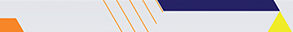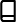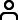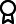Journal metrics
Acceptance rate15%
Submission to final decision42 days
Acceptance to publication64 days
CiteScore1.900
Journal Citation Indicator-
Impact Factor-

Article of the Year 2020

Mathematical Modelling and Analysis of Transmission Dynamics of Lassa FeverJournal profile

Journal of Applied Mathematics publishes original research papers and review articles in all areas of applied, computational, and industrial mathematics.Editor spotlight

Chief Editor, Professor Theodore E. Simos, is based at Ulyanovsk State Technical University, Russia. His main research interest is the numerical analysis of differential equations.Special Issues

Do you think there is an emerging area of research that really needs to be highlighted? Or an existing research area that has been overlooked or would benefit from deeper investigation? Raise the profile of a research area by leading a Special Issue.

Latest Articles

More articles
Research Article

A Stage-Structured Fishery Model for African Catfish and Nile Tilapia Feeding on Two Food Resources with Harvesting

In this paper, a fishery model for African catfish and Nile tilapia is formulated. This model is used to compare financial profit and biomass outtakes in a two-species system versus single species systems. We consider a stage-structured fish population model consisting of the aforementioned fish species together with two food resources. The model dynamics include cannibalism, predator-prey, feeding, reproduction, maturation, development, mortality, and harvesting. We prove consistency of the model in the sense that the solutions will stay bounded and nonnegative over time. Conditions for local stability of fish-free equilibrium point are established. The simulation results reveal asymptotically stable solutions with coexistence of African catfish, Nile tilapia, and two food resources. The major conclusion from our findings is that fisheries should culture both species to maximize the biomass outtake and financial profit.

Research Article

Mathematical Model and Analysis of Corruption Dynamics with Optimal Control

In this study, a deterministic mathematical model that explains the transmission dynamics of corruption is proposed and analyzed by considering social influence on honest individuals. Positivity and boundedness of solution of the model are proved and basic reproduction number is computed using the next-generation matrix method. The analysis shows that corruption-free equilibrium is locally and globally asymptotically stable whenever . Also, the endemic equilibrium point is locally and globally asymptotically stable whenever . Then, the model was extended to optimal control, and some numerical simulations with and without optimal control are also performed to verify the theoretical analysis using MATLAB. Numerical simulation of optimal control model shows that the prevention and punishment strategy is the most effective strategy to reduce the dynamic transmission of corruption.

Research Article

Numerical Solution of Three-Dimensional Transient Heat Conduction Equation in Cylindrical Coordinates

Heat equation is a partial differential equation used to describe the temperature distribution in a heat-conducting body. The implementation of a numerical solution method for heat equation can vary with the geometry of the body. In this study, a three-dimensional transient heat conduction equation was solved by approximating second-order spatial derivatives by five-point central differences in cylindrical coordinates. The stability condition of the numerical method was discussed. A MATLAB code was developed to implement the numerical method. An example was provided in order to demonstrate the method. The numerical solution by the method was in a good agreement with the exact solution for the example considered. The accuracy of the five-point central difference method was compared with that of the three-point central difference method in solving the heat equation in cylindrical coordinates. The solutions obtained by the numerical method in cylindrical coordinates were displayed in the Cartesian coordinate system graphically. The method requires relatively very small time steps for a given mesh spacing to avoid computational instability. The result of this study can provide insights to use appropriate coordinates and more accurate computational methods in solving physical problems described by partial differential equations.

Research Article

Asumu Fractional Derivative Applied to Edge Detection on SARS-COV2 Images

Edge detection consists of a set of mathematical methods which identifies the points in a digital image where image brightness changes sharply. In the traditional edge detection methods such as the first-order derivative filters, it is easy to lose image information details and the second-order derivative filters are more sensitive to noise. To overcome these problems, the methods based on the fractional differential-order filters have been proposed in the literature. This paper presents the construction and implementation of the Prewitt fractional differential filter in the Asumu definition sense for SARS-COV2 image edge detection. The experiments show that these filters can avoid noise and detect rich edge details. The experimental comparison show that the proposed method outperforms some edge detection methods. In the next paper, we are planning to improve and combine the proposed filters with artificial intelligence algorithm in order to program a training system for SARS-COV2 image classification with the aim of having a supplemental medical diagnostic.

Research Article

Applications of OHAM and MOHAM for Fractional Seventh-Order SKI Equations

In this article, a comparative study between optimal homotopy asymptotic method and multistage optimal homotopy asymptotic method is presented. These methods will be applied to obtain an approximate solution to the seventh-order Sawada-Kotera Ito equation. The results of optimal homotopy asymptotic method are compared with those of multistage optimal homotopy asymptotic method as well as with the exact solutions. The multistage optimal homotopy asymptotic method relies on optimal homotopy asymptotic method to obtain an analytic approximate solution. It actually applies optimal homotopy asymptotic method in each subinterval, and we show that it achieves better results than optimal homotopy asymptotic method over a large interval; this is one of the advantages of this method that can be used for long intervals and leads to more accurate results. As far as the authors are aware that multistage optimal homotopy asymptotic method has not been yet used to solve fractional partial differential equations of high order, we have shown that this method can be used to solve these problems. The convergence of the method is also addressed. The fractional derivatives are described in the Caputo sense.

Research Article

Effects of Drugs Reabsorption Time Delays for a Pharmacokinetics Model in Humans

A three-compartmental delay model is formulated to describe the pharmacokinetics of drugs subjected to both intravenous and oral doses with reabsorptions by the central compartment. Model dynamics are analyzed rigorously, and two equilibrium points are obtained to be locally asymptotically stable under certain conditions. Time delays used as lags in reabsorption of drugs by central compartment from other two compartments caused rebounds or peaks and fluctuations in the time profiles for amounts of drug in all the compartments. Sensitivity analysis revealed that elimination rates decrease the amounts in all compartments. Furthermore, reabsorption rates cause superimposition at the initial phases of the drug amount profiles; subsequently, the quantities decrease in compartment one and increase in compartments two and three, respectively.Journal metrics
Acceptance rate15%
Submission to final decision42 days
Acceptance to publication64 days
CiteScore1.900
Journal Citation Indicator-
Impact Factor-Author guidelinesEditorial boardDatabases and indexingAuthor guidelinesEditorial boardDatabases and indexing

Article of the Year Award: Outstanding research contributions of 2020, as selected by our Chief Editors. Read the winning articles.